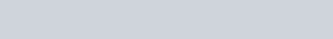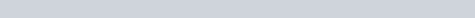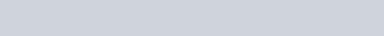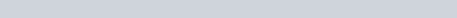Integration – Area under a graph

Integration can be used to find the area bounded by a curve y = f(x), the x-axis and the lines x=a and x=b by using the following method.However, you must be very careful in the way you use this as the following examples will show.

Area bounded by a curve when it is above the x-axis

In this tutorial I show you how to find the area bounded by a curve when it is above the x-axis.Find the area:

1.How to find the area bounded by a curve above the x-axis (tutorial 1) : ExamSolutions - youtube Video

Finding the area below the x-axis

In this tutorial I show you how to find the area bounded by a curve when it is below the x-axis.Find the area:

1.Area bounded by a curve below the x-axis (tutorial 2) : ExamSolutions - youtube Video

Finding an area above and below the x-axis

In this tutorial I show you how to find the area bounded by a curve when it is partly below the x-axis and partly above the x-axis using the following example.Find the area:

1.Area bounded by a curve above and below the x-axis (tutorial 3) : ExamSolutions - youtube Video

Finding the area bounded by two curves

In this tutorial I show you several ways of how to find the area bounded by two curves using the following example.Find the area:

1.How to find the area bounded by two curves (tutorial 4) : ExamSolutions - youtube Video